×

### Html & Html5

Introduction to Html

Html5 Grid

Html5 Graphics

Html5 Media

### Python String

String here in Python used to define unicode characters. There is no special character data type , a single character in Python is just a string of length of 1. Here in Python , string can be written in the form of single quotation or double quotation that means ‘Hello’ =”Hello”. And string can also be output too if it is used in print function.
For example:-

print("Hello World")

print('Hello World')

### Output of this Program

In above program we just gave command of printing ‘Hello World ’ by using single quotation and double quotation .
Let’s see whether it works or not :-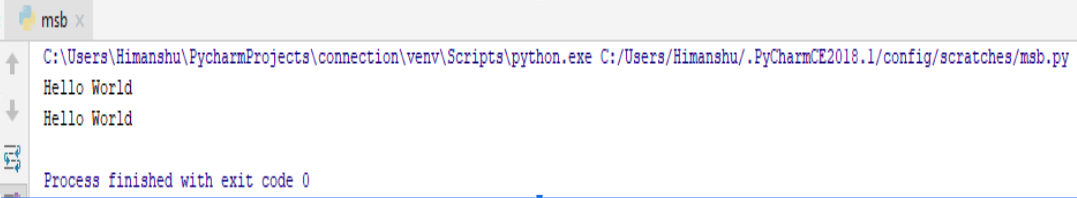So both are working that means we can use both double quotation or single quotation to use string in our program.

### Slicing in Python String

1. Python gives us the ease to select any particular character from the string if we want . For this we have to used square brackets [] to access the element. But here the first element has index or position 0.
For example :-

Get the character at position 4 of ‘Hello world’?

a="Hello world"

print(a)

### Output of this Program

As we have earlier discussed that the first character have the position or index ‘0’ . So let’s see what happens after giving command of getting the character which is having 4th position .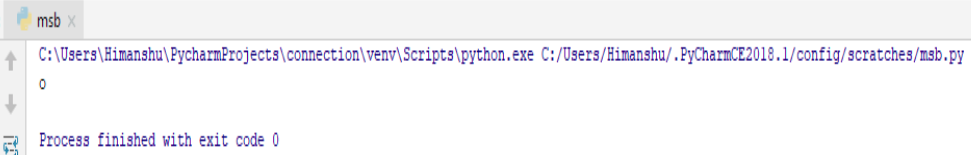So here it prints ‘O’ character because it comes on the position 4 after taking 1st character as position ‘0’

2. In Python we can also choose substring from any position to any. Let us understand this by following example :-

Get the character from position 2 to position 6 (not included)?

a="Hello world"

print(a[2:6])

### Output of this Program

Here in above program we are giving command to Python that we want the substring from the position 2 to position 6 . So let’s see what happens :-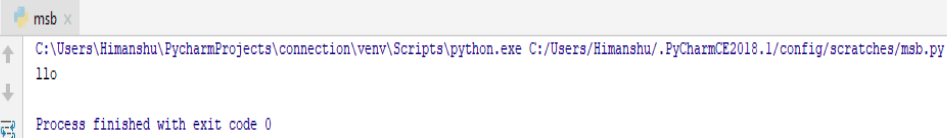So here we just got the output which comes under position 2 to position 6.It also includes spaces if there are so.

3. If in case we want to skip some character in any substring than also through the help of slicing we can do that . Let’s understand this by example :-

Get the elements by skipping 1 character from the string

a="Hello world"

print(a[0:12:2])

### Output of this Program

Here in above program we just gave command to our Python interpreter that from the word “Hello word ” we want to extract the word by skipping the next word. So let’s see…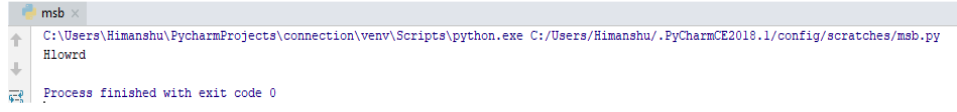So here as we can see , we are getting the words by skipping the next word. Like this we can skip as much words we want.

### Some built-in methods of String in Python

Methods are basically the function which we want to perform in our coding . In Python there are some inbuilt methods are there for String .
So let’s see some of them :-

1. The len() method returns the length of a string

a = "Hello world"

print(len(a))

### Output of this ProgramSo here we just get the length of our string.

2. The lower() method returns the whole string in lower letters.

a = "Hello world"

print(a.lower())

### Output of this ProgramSo here we just get our whole string in lower letters.

3. The type () method returns basically the type of the data type.

a = "Hello world"

print(type(a))

### Output of this Program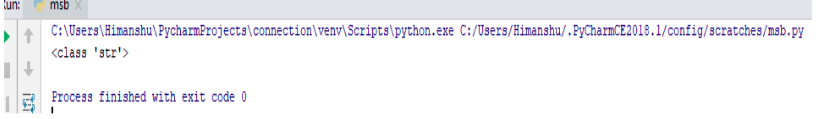So here we just get the data type .

### Some more methods and their functionality

So here we are enlisting some methods and their description which we can use in Python.

METHODDESCRIPTION
capitalize() Converts the first character to uppercase
casefold() Converts string into lower case
center() Returns a centered string
count() Returns the number of times a specified value occurs in a string
encode() Returns an encoded version of the string
endswith() Returns true if the string ends with the specified value
expandtabs() Set the tab size of string
find() Searches the string for a specified value and returns the position of where it was found
format() Formats specified values in a string
format_map() Formats specified values in a string
index()) Searches the string for a specified value and returns the position of where it was found
isalnum() Returns True if all characters in the string are alphanumeric
isalpha() Returns True if all characters in the string are in the alphabet
isdecimal() Returns True if all characters in the string are decimals
isdigit() Returns True if all characters in the string are digits
isidentifier() Returns True if the string is an identifier
islower() Returns True if all characters in the string are in lower case
isnumeric() Returns True if all characters in the string are numeric
isprintable() Returns True if all characters in the string are printable
isspace() Returns True if all characters in the string are whitespaces
istitle() Returns True if the string follows the rules of a title
isupper() Returns True if all characters in the string are in upper case
join() Joins the elements of an iterable to the end of the string
|just() Returns a left justified version of the string
lower() Converts a string into lower case
|strip() Returns a left trim version of the string
maketrans() Returns a translation table to be used in translations
partition() Returns a tuple where the string is divided into three parts
replace() Returns a string where a specified value is replaced with a specified value
rfind() Searches the string for a specified value and returns the last position of where it was found
rindex() Searches the string for a specified value and returns the last position of where it was found
rjust() Returns a right justified version of the string
rpartition() Returns a tuple where the string is divided into three parts
rsplit() Splits the string at the specified separator, and returns a list
rstrip() Returns a right trim version of the string
split() Splits the string at the specified separator, and returns a list
splitlines() Splits the string at line breaks and returns a list
startswith() Returns true if the string starts with the specified value
strip() Returns a trimmed version of the string
swapcase() Swaps cases, lower case becomes upper case and vice versa
title() Converts the first character of each word to uppercase
translate() Returns a translated string
upper() Converts a string into upper case
zfill() Fills the string with a specified number of 0 values at the beginning

python training insitute| Best IT Training classes in Gurgaon | python training